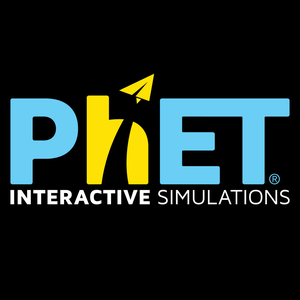# Trig Tour

Source: UC Boulder PhET

Student Price: FREE

Take a tour of trigonometry using degrees or radians! Look for patterns in the values and on the graph when you change the value of theta.

# Trig Tour: Simulation

• Trigonometry
• Unit circle
• Sines
• Cosines
• Tangents

### Description

Take a tour of trigonometry using degrees or radians! Look for patterns in the values and on the graph when you change the value of theta. Compare the graphs of sine, cosine, and tangent.

### Sample Learning Goals

• Define trig functions for negative angles and angles greater than 90 degrees.
• Translate between multiple representations of trig functions: as sides of a right triangle inscribed in a unit circle, graph of the function vs. angle, and numerical values of the function.
• Deduce the sign (+, -, 0) of a trig function for any given angle without a calculator using the unit circle concept.
• Estimate the value of trig functions for any given angle without a calculator using the unit circle concept.
• Define exact trig functions for special angles using degrees or radians for angle measures.